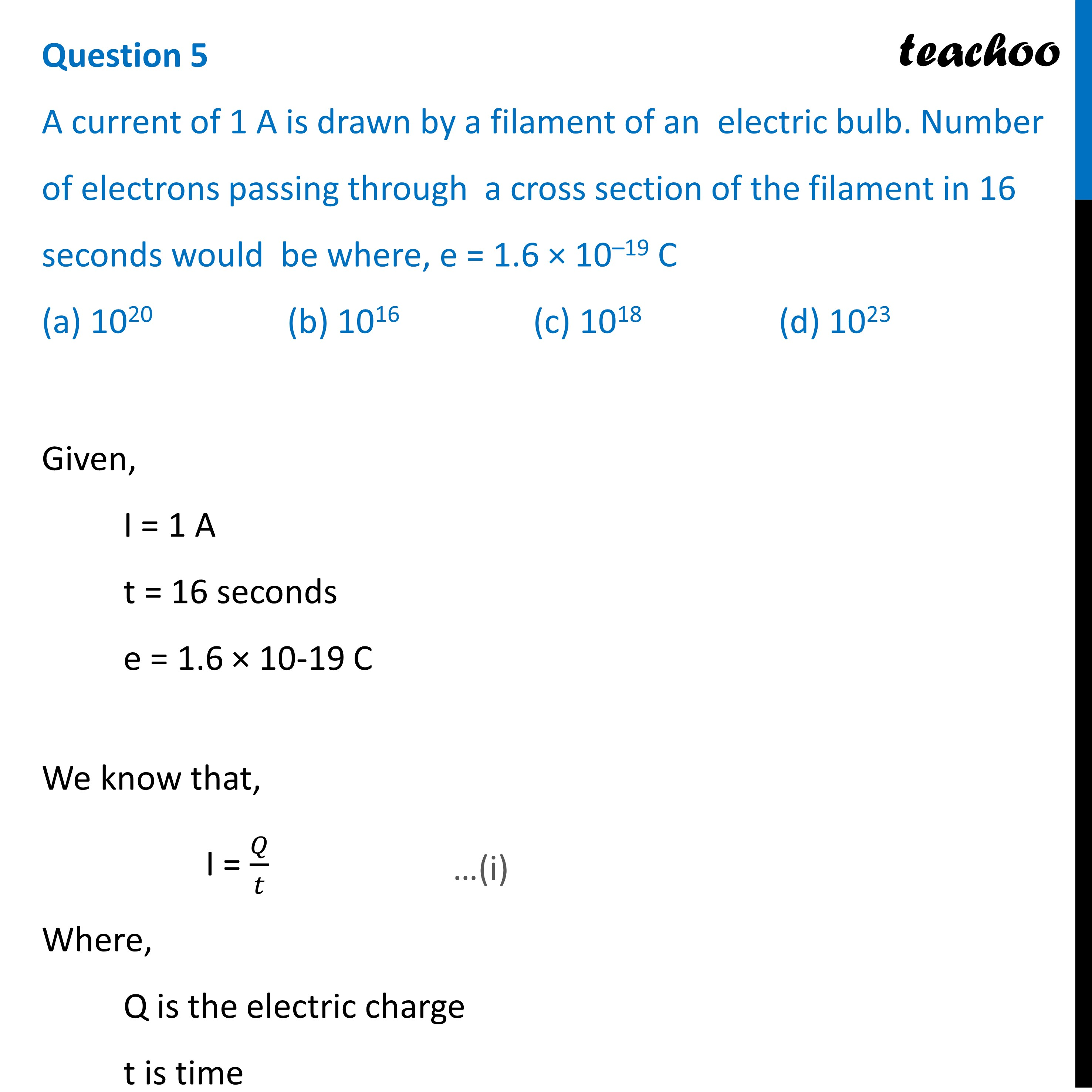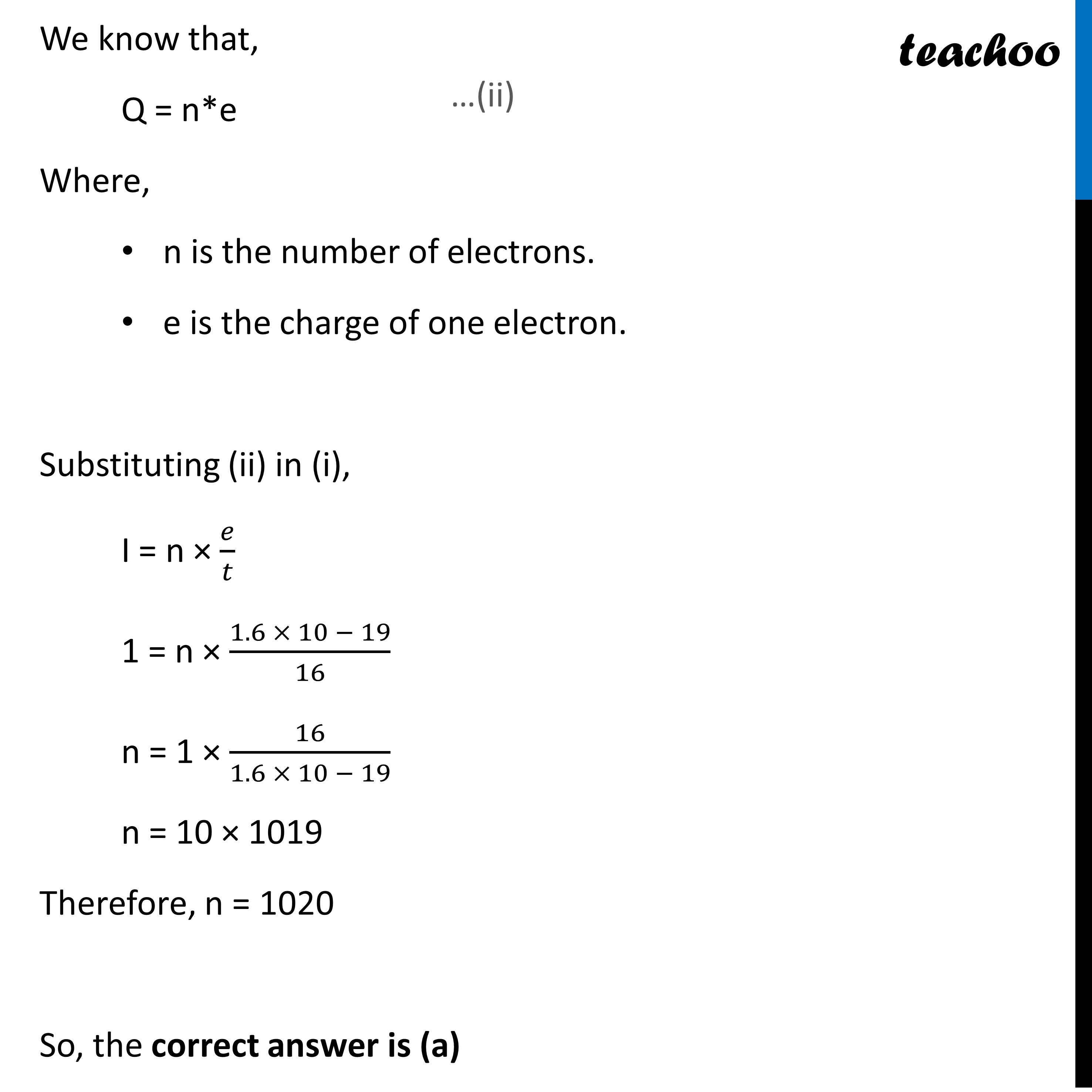MCQs from (Past Year Papers & NCERT Exemplar)

Class 10
Chapter 12 Class 10 - Electricity

## (d) 10 23Given,

• I = 1 A
• t = 16 seconds
• e = 1.6 × 10 -19 C

We know that,

I = Q / t             —------(i)

Where,

• Q is the electric charge
• t is time

We know that,

Q = n*e           —---------(ii)

Where,

• n is the number of electrons.
• e is the charge of one electron.

Substituting (ii) in (i),

I = n*e / t

1 = n * 1.6 × 10 -19  / 16

n = 1 * 16 / 1.6 × 10 -19

n = 10 * 10 19

Therefore, n = 10 20

So, the correct answer is (a).

Learn in your speed, with individual attention - Teachoo Maths 1-on-1 Class

### Transcript

Question 5 A current of 1 A is drawn by a filament of an electric bulb. Number of electrons passing through a cross section of the filament in 16 seconds would be where, e = 1.6 × 10–19 C (a) 1020 (b) 1016 (c) 1018 (d) 1023Given, I = 1 A t = 16 seconds e = 1.6 × 10-19 C We know that, I = 𝑄/𝑡 Where, Q is the electric charge t is time We know that, Q = n*e Where, n is the number of electrons. e is the charge of one electron. Substituting (ii) in (i), I = n × 𝑒/𝑡 1 = n × (1.6 × 10 − 19)/16 n = 1 × 16/(1.6 × 10 − 19) n = 10 × 1019 Therefore, n = 1020 So, the correct answer is (a)